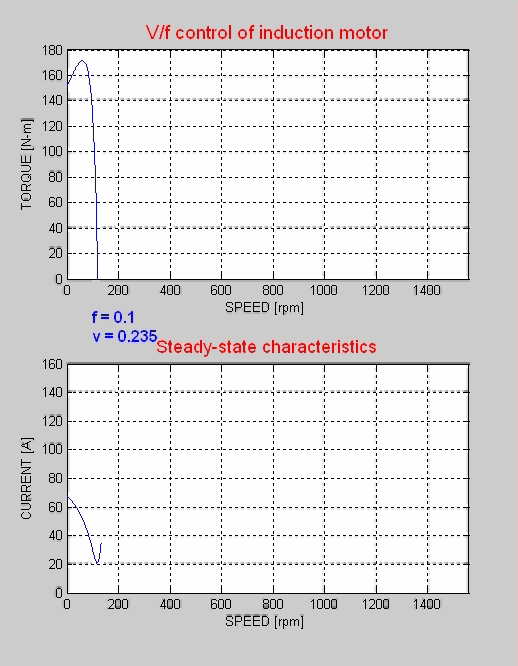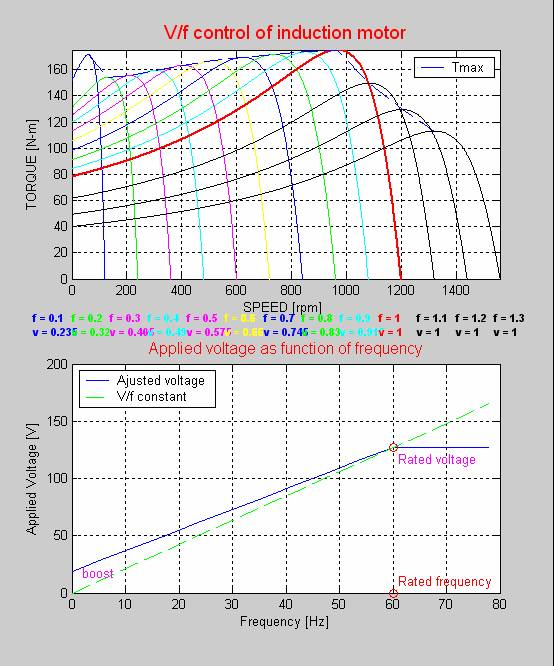﻿ Volts/Hz speed control of an induction motor

VOLT PER HZ SPEED CONTROL OF AN INDUCTION MOTOR

The speed of an induction motor can be easily controlled by varying the frequency of the 3-phase supply; however, to maintain a constant (rated) flux density, the applied voltage must also be changed in the same proportion as the frequency (as dictated by Faraday’s law).This speed control method is known as Volts per Hz. Above rated speed, the applied voltage is usually kept constant at rated value; this operation is referred to as constant HP. At low frequencies (i.e. speeds), the voltage must be boosted in order to compensate for the effects of the stator resistance.